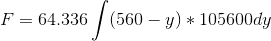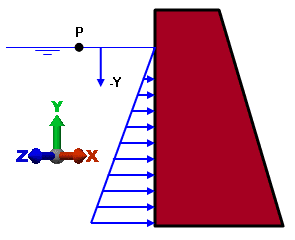# Help Calculating Pressure of Seawater against a flat surface

Hi, I'm trying to figure out the pressure that seawater would exert against a vertical surface (on average) over a large area but I am messing up somewhere. The height of the rectangle is 560 ft and the length is 20 miles, or 105,600 ft, giving an area of 2.12 mi². The weight of the water in question is 4,735 megatons (the density of the water due to salinity is 64.336 lbs/ft³).

I attempted to follow the outline linked below, but I seem to be running afoul somewhere. Thanks for any help you are willing to provide.

Some of the math above is wrong, but the formula I am using is:where my integral is from 0 to 560.

SteamKing
Staff Emeritus
Homework Helper
Some of the math above is wrong, but the formula I am using is:where my integral is from 0 to 560.
Do you want to calculate the pressure acting on the wall, or the total force, which is what F is?

According to Pascal's Law, P = ρ ⋅ g ⋅ h, where ρ ⋅ g = 64.336 lbf / ft3

At the top of your wall, P = 0 lbf / ft2, while at a depth of 560 ft, P = 560 ⋅ 64.336 = 36,028 lbf / ft2 = 250 p.s.i.

The total force acting on a strip of wall 1 foot wide is 64.336 (560 y - y2/2), evaluated for 0 ≤ y ≤ 560 ft, or F = 10,087,885 lbf / ft. width

For a wall 20 statute miles long, the force F would be 10,087,885 lbs / ft. * 105,600 ft. = 1.065 × 1012 lbf = 532,640,628 tons (1 ton = 2000 lbf)

Do you want to calculate the pressure acting on the wall, or the total force, which is what F is?

According to Pascal's Law, P = ρ ⋅ g ⋅ h, where ρ ⋅ g = 64.336 lbf / ft3

At the top of your wall, P = 0 lbf / ft2, while at a depth of 560 ft, P = 560 ⋅ 64.336 = 36,028 lbf / ft2 = 250 p.s.i.

The total force acting on a strip of wall 1 foot wide is 64.336 (560 y - y2/2), evaluated for 0 ≤ y ≤ 560 ft, or F = 10,087,885 lbf / ft. width

For a wall 20 statute miles long, the force F would be 10,087,885 lbs / ft. * 105,600 ft. = 1.065 × 1012 lbf = 532,640,628 tons (1 ton = 2000 lbf)

I want to calculate the pressure acting on the wall; it is 250 PSI at the base? What would the average PSI be? Thanks again for the help.

SteamKing
Staff Emeritus
Homework Helper
I want to calculate the pressure acting on the wall; it is 250 PSI at the base? What would the average PSI be? Thanks again for the help.
0 at the top, 250 psi at the bottom, 125 psi average.

•Chestermiller
since you have only given the pressure at the top 0psi and at the bottom 250psi
then the psi on the wall at any given point along the wall at a depth of 280 ft
would be 1/2 of 250psi
250psi/2=125psi

the psi of a standing column of water can be found by multiplying the weight of
1 cu in of the water x the height of the column of water in inches.

you set the psi at the base to 250psi
and the height of the column of water to 560 ft
(250psi/560ft)/12 inches = .037202 lbs/cu in

.037202 lbs/cu in * ((560ft / 2)*12)=124.9987psi

SteamKing
Staff Emeritus
Homework Helper
since you have only given the pressure at the top 0psi and at the bottom 250psi
then the psi on the wall at any given point along the wall at a depth of 280 ft
would be 1/2 of 250psi
250psi/2=125psi

the psi of a standing column of water can be found by multiplying the weight of
1 cu in of the water x the height of the column of water in inches.

you set the psi at the base to 250psi
and the height of the column of water to 560 ft
(250psi/560ft)/12 inches = .037202 lbs/cu in

.037202 lbs/cu in * ((560ft / 2)*12)=124.9987psi
Or the weight of 1 cubic foot of water by the depth of water in feet. Same difference.

Or the weight of 1 cubic foot of water by the depth of water in feet. Same difference.

actually theres a really big difference.

that would deliver an answer of 17999.82 lbs vertical pressure per horizontal square ft.

there is no way that he could use that number unless he divided it by the 144 sq inches
in the horizontal square foot.
which results in 124.998 psi

he was wanting the average pressure that acts horizontally against the wall.

Last edited:
SteamKing
Staff Emeritus
Homework Helper
actually theres a really big difference.

that would deliver an answer of 8035.632 lbs vertical pressure per horizontal square ft.

there is no way that he could use that number.

he was wanting the average pressure that acts horizontally against the wall.
It's not clear what you are talking about.

Pascal's Law works the same for cubic feet as it does for cubic inches.

If the depth of water is 560 feet and the weight density of seawater is 64.336 lbf / ft3, then the hydrostatic pressure at 560 feet is

P = 560 ⋅ 64.336 = 36,028 lbf/ft2

Since there are 144 in2 in one square foot, this pressure is equivalent to 36,028 / 144 = 250 p.s.i. at a depth of 560 feet.

The hydrostatic pressure at the surface is P = 0 p.s.i. by definition, therefore the average hydrostatic pressure = 250 p.s.i. / 2 = 125 p.s.i.

The pressure profile is triangular w.r.t. depth, like so:But let's check the numbers anyway.

1 cu. ft = 1728 cu. in.

1 cu.ft. of seawater weighs 64.336 lbf, so 1 cu.in. seawater weighs 64.336 / 1728 = 0.0372 lbf

At a depth of 560 feet = 6720 inches, the hydrostatic pressure P = 0.0372 ⋅ 6720 = 250 lbf / in2 = 250 p.s.i.

Looks like the same numbers to me.

I was pointing out that he wouldnt want to simply multiply the weight of
1 cu ft of sea water by the depth of the sea water @ 280 ft to find the average pressure
of the sea water that acts against the wall.

and that he would need to divide the result of your suggested math by 144 to find the average psi.